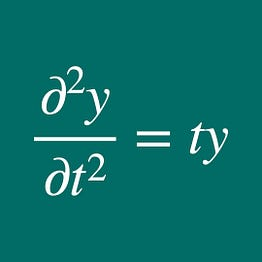# An easy example of STFT

Suppose we have an image of a wave:

This wave is inverse transformed to one in the frequency coordinates. To do so, use short time fourier transform. Here we use method signal.stft from scipy:

`from scipy import signal  f0, t0, Zxx=signal.stft(original_wave, fs=1 )`

where fs is the sampling frequency. Then plot it

`ax.plot(f0, Zxx, lw=2.5)`

# A Decision Tree Code

Last year I wrote a blog article regarding decision tree. I’ve put relating code here.

# Stacked bar chart in seaborn

The codes are here.

# Posting messages to Salesforce’s Chatter

## simple_salesforce allows us to do it

API simple_salesforce allows us to access Salesforce. And we can use it to post a message to Salesforce’s Chatter.

`from simple_salesforce import Salesforceimport requests import pandas as pdimport csv import requestsfrom io import StringIOimport getpassimport jsonimport datetime`

`sf0=Salesforce(username=USERNAME, password=PASSWORD, security_token=SECURITY_TOKEN)`

Then, we use restful method and json to post a message to Chatter.

`message_a="Awesome! A message was posted at " + datetime.datetime.now().strftime('%Y-%m-%d %H:%M:%S')user_id=USER_IDbody0 = { "body":     {"messageSegments": [        {"type": "Text", "text": message_a},         {"type": "Mention", "id": user_id}    ]},     "feedElementType": "FeedItem", "subjectId": "me"}zz_rest0=sf0.restful(r'chatter/feed-elements/', method='POST', data=json.dumps(body0))`

Here user_id is the id of the user whom we send a message.

# To avoid confusion about the direction of an axis in Python Pandas

## Get a basic insight

In python pandas, axis=0 means that a dataframe (table) is processed in the way that a row is a building block. For instance, you use pandas.concat to stack rows:

# A simple code for Latent Dirichlet Allocation

An easy code to carry out LDA

First we import the following libraries:

`import MeCabfrom sklearn.feature_extraction.text import CountVectorizer from sklearn.decomposition import LatentDirichletAllocation`

Here, MeCab is a library to tokenize Japanese texts. Then, using CountVectorizer, we make a table to count the number of appearance for each word.

`num_topics=6print(len(text0))cnt_vec…`

# Easy Explanation about Deep Learning

Suppose a neural network consists of 3 layers: input, hidden and output layers. Each layers are linked to each other via a neuron-like object, and the information propagates from the input layer to the output.

Each layer has parameters, and in this case parameters are V, W, b, c. What the neural network learns means that the parameters are optimized such that the error is minimized. The optimization is done through iteration. This is the main process of deep learning.

# Easy implementation of BoW

Bag-of-words (BoW) is a simple way to represent a collection of documents under the assumption that each document is represented by a distribution of words. In the model, the number of times that words appear in each document of a collection is counted. BoW is often used in document classification.

And its implementation is easy; you only use scikit-learn and nltk (provided the word set is in English.) First, you use nltk to have:

Secondly, you use scikit-learn to have:

# An interim solution to a Tableau data connection error

Suppose you use Tableau on macOS & try to connect to a data source (eg .xlsx file). And if you get an error message like

`An error occurred while communicating with Tableau Data ExtractBad Connection: Tableau could not connect to the data source.Error Code: ****** Could not start Hyper: Failed to start a new Hyper instance.Caused by:The Hyper server process exited during startup with exit code: 1 d.log”, 514): Operation not permitted `

then you can solve the problem in a simple way (although the solution is not permanent).

The solution is to start Tableau from a terminal: for example if you use Tableau Public, you type:

`/Applications/Tableau\ Public.app/Contents/MacOS/Tableau\ Public`

Then you can connect to a data source.

# Manually set up nltk-related data to start the toolkit

`>>> import nltk>>> nltk.download('punkt')`
`[nltk_data] Error loading punkt: <urlopen error [SSL:[nltk_data]     CERTIFICATE_VERIFY_FAILED] certificate verify failed:[nltk_data]…`